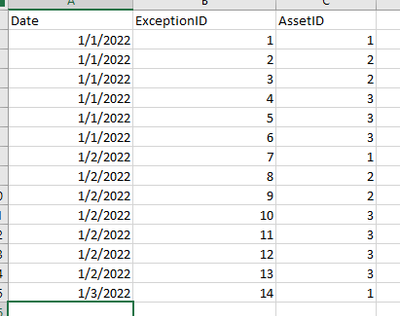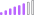cancel
Showing results for
Did you mean:Helper I

## record with max value by day

I have a dataset below that has multiple records on a given day.  What I would like to do is find the AssetID with the max number (count) of ExceptionID by day....I was able to use groupby and rollup records by Date and AssetID to get summary table as below

Rollup = GROUPBY(transactions, transactions[Date],transactions[AssetID],
"Exception Count"COUNTX(CURRENTGROUP(),transactions[ExceptionID]))Now I want to simply pul out the max exception count per day... so for each day there should be 1 record with exception count and Asset ID...

Do I simply add a second filter to get max, by day,  where day can be a part of filter context?

7 REPLIES 7Super User

1. Create the rollup table in DAX like you posted above.

2. Add column using this syntax

``````"Max Exception in a day" =
CALCULATE( MAX([Exception Count]),
FILTER('Rollup Table', 'Rollup Table'[transactions_date] = EARLIER('Rollup Table'[transactions_date])))``````

Note: If you are using total, it will give sum of these.

3. (My preference) Create a measure

``````"Max Exception" =
var _sel = SELECTEDVALUE('Rollup Table'[transactions_date])

Max in a day = IF(
HASONEVALUE('Rollup Table'[transactions_date]),
Maxx(
Filter(all('Rollup Table'), [transactions_date] = _sel),
'Rollup Table'[Exception Count])
, Max('Rollup Table'[Exception Count])
)``````Post Patron

Hi @sbuster
you Could try this one,

Measure=Maxx(values(transactions[Date]),Calculate(Count(transactions[ExceptionID]))

Thanks,
ThennarasuSuper User

You could try

``````Top exceptions by day =
GENERATE (
VALUES ( 'Table'[Date] ),
TOPN (
1,
CALCULATETABLE (
GROUPBY (
transactions,
transactions[AssetID],
"Exception Count", COUNTX ( CURRENTGROUP (), transactions[ExceptionID] )
)
),
"Exception Count"
)
)
``````Helper I

This formula simply returns the same result as my rollup table shown above.  The next step is to produce 1 record per day, where that record should be the one with the max exception count.  So basically 1 record for every day, with the assetid and the exception count (which is the result of finding the max exception count).Helper I

I was able to solve this problem by finding this solution.  Thanks to everyone for your suggestions.Community Champion

hi @sbuster

how about plot a table visual with three columns in Rollup table and choose Max for Exception Count column?Helper I

This still returns multiple records for the same day.